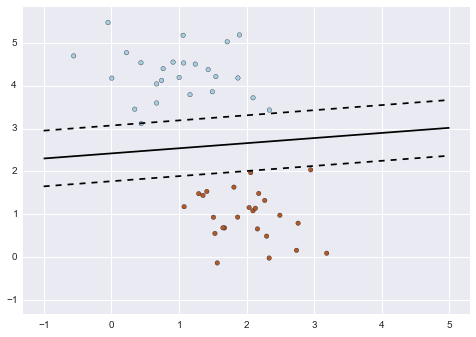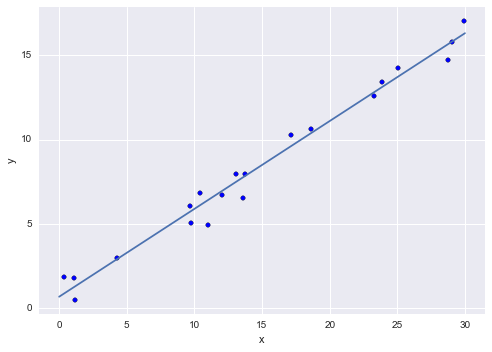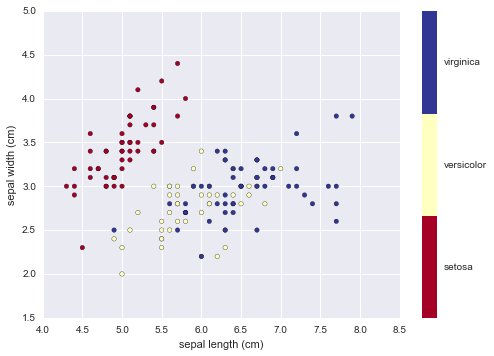This notebook was put together by [Jake Vanderplas](http://www.vanderplas.com) for PyCon 2015. Source and license info is on [GitHub](https://github.com/jakevdp/sklearn_pycon2015/).

# Introduction to Scikit-Learn: Machine Learning with Python¶

This session will cover the basics of Scikit-Learn, a popular package containing a collection of tools for machine learning written in Python. See more at http://scikit-learn.org.

## Outline¶

Main Goal: To introduce the central concepts of machine learning, and how they can be applied in Python using the Scikit-learn Package.

• Definition of machine learning
• Data representation in scikit-learn
• Introduction to the Scikit-learn API

Scikit-Learn is a Python package designed to give access to well-known machine learning algorithms within Python code, through a clean, well-thought-out API. It has been built by hundreds of contributors from around the world, and is used across industry and academia.

Scikit-Learn is built upon Python's NumPy (Numerical Python) and SciPy (Scientific Python) libraries, which enable efficient in-core numerical and scientific computation within Python. As such, scikit-learn is not specifically designed for extremely large datasets, though there is some work in this area.

For this short introduction, I'm going to stick to questions of in-core processing of small to medium datasets with Scikit-learn.

## What is Machine Learning?¶

In this section we will begin to explore the basic principles of machine learning. Machine Learning is about building programs with tunable parameters (typically an array of floating point values) that are adjusted automatically so as to improve their behavior by adapting to previously seen data.

Machine Learning can be considered a subfield of Artificial Intelligence since those algorithms can be seen as building blocks to make computers learn to behave more intelligently by somehow generalizing rather that just storing and retrieving data items like a database system would do.

We'll take a look at two very simple machine learning tasks here. The first is a classification task: the figure shows a collection of two-dimensional data, colored according to two different class labels. A classification algorithm may be used to draw a dividing boundary between the two clusters of points:

In :
%matplotlib inline

# set seaborn plot defaults.
# This can be safely commented out
import seaborn; seaborn.set()

In :
# Import the example plot from the figures directory
from fig_code import plot_sgd_separator
plot_sgd_separator()This may seem like a trivial task, but it is a simple version of a very important concept. By drawing this separating line, we have learned a model which can generalize to new data: if you were to drop another point onto the plane which is unlabeled, this algorithm could now predict whether it's a blue or a red point.

If you'd like to see the source code used to generate this, you can either open the code in the figures directory, or you can load the code using the %load magic command:

In :
#Uncomment the %load command to load the contents of the file


The next simple task we'll look at is a regression task: a simple best-fit line to a set of data:

In :
from fig_code import plot_linear_regression
plot_linear_regression()Again, this is an example of fitting a model to data, such that the model can make generalizations about new data. The model has been learned from the training data, and can be used to predict the result of test data: here, we might be given an x-value, and the model would allow us to predict the y value. Again, this might seem like a trivial problem, but it is a basic example of a type of operation that is fundamental to machine learning tasks.

## Representation of Data in Scikit-learn¶

Machine learning is about creating models from data: for that reason, we'll start by discussing how data can be represented in order to be understood by the computer. Along with this, we'll build on our matplotlib examples from the previous section and show some examples of how to visualize data.

Most machine learning algorithms implemented in scikit-learn expect data to be stored in a two-dimensional array or matrix. The arrays can be either numpy arrays, or in some cases scipy.sparse matrices. The size of the array is expected to be [n_samples, n_features]

• n_samples: The number of samples: each sample is an item to process (e.g. classify). A sample can be a document, a picture, a sound, a video, an astronomical object, a row in database or CSV file, or whatever you can describe with a fixed set of quantitative traits.
• n_features: The number of features or distinct traits that can be used to describe each item in a quantitative manner. Features are generally real-valued, but may be boolean or discrete-valued in some cases.

The number of features must be fixed in advance. However it can be very high dimensional (e.g. millions of features) with most of them being zeros for a given sample. This is a case where scipy.sparse matrices can be useful, in that they are much more memory-efficient than numpy arrays.

## A Simple Example: the Iris Dataset¶

As an example of a simple dataset, we're going to take a look at the iris data stored by scikit-learn. The data consists of measurements of three different species of irises. There are three species of iris in the dataset, which we can picture here:

In :
from IPython.core.display import Image, display
display(Image(filename='images/iris_setosa.jpg'))
print("Iris Setosa\n")

display(Image(filename='images/iris_versicolor.jpg'))
print("Iris Versicolor\n")

display(Image(filename='images/iris_virginica.jpg'))
print("Iris Virginica")Iris SetosaIris VersicolorIris Virginica


### Quick Question:¶

If we want to design an algorithm to recognize iris species, what might the data be?

Remember: we need a 2D array of size [n_samples x n_features].

• What would the n_samples refer to?

• What might the n_features refer to?

Remember that there must be a fixed number of features for each sample, and feature number i must be a similar kind of quantity for each sample.

Scikit-learn has a very straightforward set of data on these iris species. The data consist of the following:

• Features in the Iris dataset:

1. sepal length in cm
2. sepal width in cm
3. petal length in cm
4. petal width in cm
• Target classes to predict:

1. Iris Setosa
2. Iris Versicolour
3. Iris Virginica

scikit-learn embeds a copy of the iris CSV file along with a helper function to load it into numpy arrays:

In :
from sklearn.datasets import load_iris

In :
iris.keys()

Out:
dict_keys(['target_names', 'DESCR', 'data', 'target', 'feature_names'])
In :
n_samples, n_features = iris.data.shape
print((n_samples, n_features))
print(iris.data)

(150, 4)
[ 5.1  3.5  1.4  0.2]

In :
print(iris.data.shape)
print(iris.target.shape)

(150, 4)
(150,)

In :
print(iris.target)

[0 0 0 0 0 0 0 0 0 0 0 0 0 0 0 0 0 0 0 0 0 0 0 0 0 0 0 0 0 0 0 0 0 0 0 0 0
0 0 0 0 0 0 0 0 0 0 0 0 0 1 1 1 1 1 1 1 1 1 1 1 1 1 1 1 1 1 1 1 1 1 1 1 1
1 1 1 1 1 1 1 1 1 1 1 1 1 1 1 1 1 1 1 1 1 1 1 1 1 1 2 2 2 2 2 2 2 2 2 2 2
2 2 2 2 2 2 2 2 2 2 2 2 2 2 2 2 2 2 2 2 2 2 2 2 2 2 2 2 2 2 2 2 2 2 2 2 2
2 2]

In :
print(iris.target_names)

['setosa' 'versicolor' 'virginica']


This data is four dimensional, but we can visualize two of the dimensions at a time using a simple scatter-plot:

In :
import numpy as np
import matplotlib.pyplot as plt

x_index = 0
y_index = 1

# this formatter will label the colorbar with the correct target names
formatter = plt.FuncFormatter(lambda i, *args: iris.target_names[int(i)])

plt.scatter(iris.data[:, x_index], iris.data[:, y_index],
c=iris.target, cmap=plt.cm.get_cmap('RdYlBu', 3))
plt.colorbar(ticks=[0, 1, 2], format=formatter)
plt.clim(-0.5, 2.5)
plt.xlabel(iris.feature_names[x_index])
plt.ylabel(iris.feature_names[y_index]);### Quick Exercise:¶

Change x_index and y_index in the above script and find a combination of two parameters which maximally separate the three classes.

This exercise is a preview of dimensionality reduction, which we'll see later.

## Other Available Data¶

They come in three flavors:

• Packaged Data: these small datasets are packaged with the scikit-learn installation, and can be downloaded using the tools in sklearn.datasets.load_*
• Downloadable Data: these larger datasets are available for download, and scikit-learn includes tools which streamline this process. These tools can be found in sklearn.datasets.fetch_*
• Generated Data: there are several datasets which are generated from models based on a random seed. These are available in the sklearn.datasets.make_*

You can explore the available dataset loaders, fetchers, and generators using IPython's tab-completion functionality. After importing the datasets submodule from sklearn, type

datasets.load_ + TAB



or

datasets.fetch_ + TAB



or

datasets.make_ + TAB



to see a list of available functions.

In :
from sklearn import datasets

In :
# Type datasets.fetch_<TAB> or datasets.load_<TAB> in IPython to see all possibilities

# datasets.fetch_

In :
# datasets.load_


In the next section, we'll use some of these datasets and take a look at the basic principles of machine learning.